Get inspired by the success stories of our students in IIT JAM MS, ISI  MStat, CMI MSc DS.  Learn More

# ISI MStat PSB 2009 Problem 3 | Gamma is not abNormalThis is a very simple but beautiful sample problem from ISI MStat PSB 2009 Problem 3. It is based on recognizing density function and then using CLT. Try it !

## Problem- ISI MStat PSB 2009 Problem 3

Using and appropriate probability distribution or otherwise show that,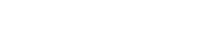.

### Prerequisites

Gamma Distribution

Central Limit Theorem

Normal Distribution

## Solution :

Here all we need is to recognize the structure of the integrand. Look, that here, the integrand is integrated over the non-negative real numbers. Now, event though here it is not mentioned explicitly that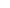is a random variable, we can assumeto be some value taken by a random variable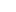. After all we can find randomness anywhere and everywhere !!

Now observe that the integrand has a structure which is very identical to the density function of gamma random variable with parametersande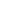. So, if we assume thatis a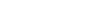, then our limiting integral transforms to,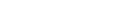.

Now, we know that if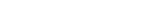, then its mean and variance both are.

So, as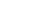,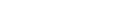, by Central Limit Theorem.

Hence,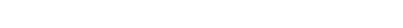. [ here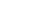is the cdf of Normal at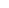.]

Hence proved !!

## Food For Thought

Can, you do the proof under the "Otherwise" condition !!

Give it a try !!

## Subscribe to Cheenta at Youtube

This is a very simple but beautiful sample problem from ISI MStat PSB 2009 Problem 3. It is based on recognizing density function and then using CLT. Try it !

## Problem- ISI MStat PSB 2009 Problem 3

Using and appropriate probability distribution or otherwise show that,.

### Prerequisites

Gamma Distribution

Central Limit Theorem

Normal Distribution

## Solution :

Here all we need is to recognize the structure of the integrand. Look, that here, the integrand is integrated over the non-negative real numbers. Now, event though here it is not mentioned explicitly thatis a random variable, we can assumeto be some value taken by a random variable. After all we can find randomness anywhere and everywhere !!

Now observe that the integrand has a structure which is very identical to the density function of gamma random variable with parametersande. So, if we assume thatis a, then our limiting integral transforms to,.

Now, we know that if, then its mean and variance both are.

So, as,, by Central Limit Theorem.

Hence,. [ hereis the cdf of Normal at.]

Hence proved !!

## Food For Thought

Can, you do the proof under the "Otherwise" condition !!

Give it a try !!

## Subscribe to Cheenta at Youtube

This site uses Akismet to reduce spam. Learn how your comment data is processed.

### Knowledge Partner